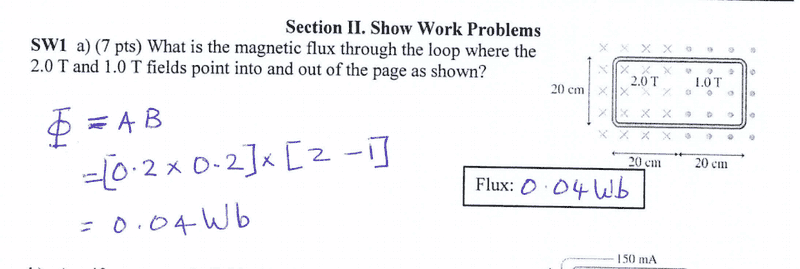# Magnetic flux through a loop

• ATT182

## Homework Statement## Homework Equations

Flux = DeltaB * Area

## The Attempt at a Solution

: Since the magnetic fields are going in opposite directions through the loop, I assumed that they would have different signs, so I chose 2.0 T to be positive and 1.0 to be negative, so when calculating the change in magnetic field, I did -1.0-2.0 = -3.0. Why is this not the case? Thanks![/B]

Last edited:

Welcome to PF!

so when calculating the change in magnetic field, I did -1.0-2.0 = -3.0. Why is this not the case? Thanks!

You are not asked to find a change in flux. You just need to find the total flux through the entire loop.

Imagine dividing the loop into left and right halves.

The change in field with respect to spatial variable is indeed -3 as we move from right to left, BUT

Faraday's law wants us to calculate the change in flux with respect to the time variable.

Welcome to PF!

You are not asked to find a change in flux. You just need to find the total flux through the entire loop.

Imagine dividing the loop into left and right halves.
Thank you! I'm starting to see it, but isn't the flux due to a change in magnetic field? So I'm still not so sure why you wouldn't choose one to be negative. Is this because flux is a scalar quantity?

isn't the flux due to a change in magnetic field?
Flux is essentially just the number of magnetic field lines piercing through the loop. Field lines in one direction count positive while field lines in the opposite direction count negative.

If the magnetic field changes, or if the size of the loop changes, or if the orientation of the loop changes, then the flux through the loop can change. A change in flux induces an emf in the loop.

But this problem is not concerned with emf or with a change in flux. It just asks for the flux for the situation shown in the figure.

•ATT182